Authorized Online Retailers:

Introduction

In this lesson, we’ll introduce how to use Raspberry Pi to get data from DHT11 Temperature and humidity sensor module .

Hardware Preparation
1 * Raspberry Pi
1 * DHT11
Jumper wires
1 * T-Extension Board with 40-Pin Cable(Optional)

Software Preparation
Note: In this lesson, we remotely control raspberry pi via PuTTy on PC. To learn how to config raspberry pi, please visit lesson 1: getting started with raspberry pi.

Experimental Principle
DHT11 module is a temperature and humidity sensor which has 3 pins: VCC, GND and DATA. This module adopts OneWire protocol to communicate. At the beginning of communication process, DATA pin sends signal to DHT11, and then DHT11 receives the signal and sends answer signal to host, at last, the host receives answer signal and starts to receive 40 bit Temperature and humidity. (8 bit integer part of humidity + 8 bit decimal part of humidity + 8 bit integer part of temperature + 8 bit decimal part of  temperature + 8 bit checksum)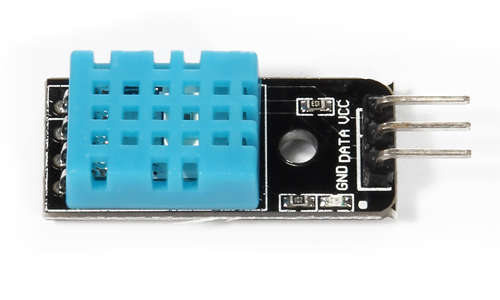Schematic diagram of DHT11Schematic diagram of DHT11 with raspberry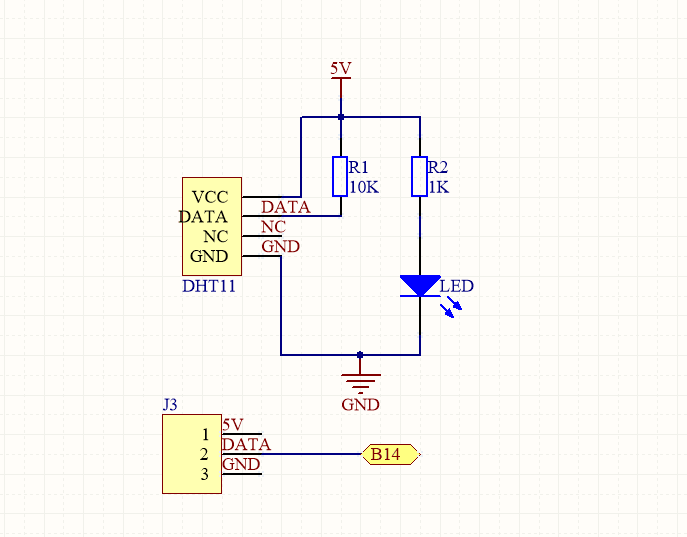B14 for in above graph means BCM GPIO#14(TXD) or Physical pin#8 or wiringPi#15
Note: B means BCM(Broadcom pin number). If you don’t know what is BCM pin#, Physical pin#, wiringPi#, please review our lesson 3: Introduction Of Raspberry Pi GPIOLearn more about GPIO of raspberry pi, please review our lesson 2: Introduction Of Raspberry Pi GPIO

Connection Graph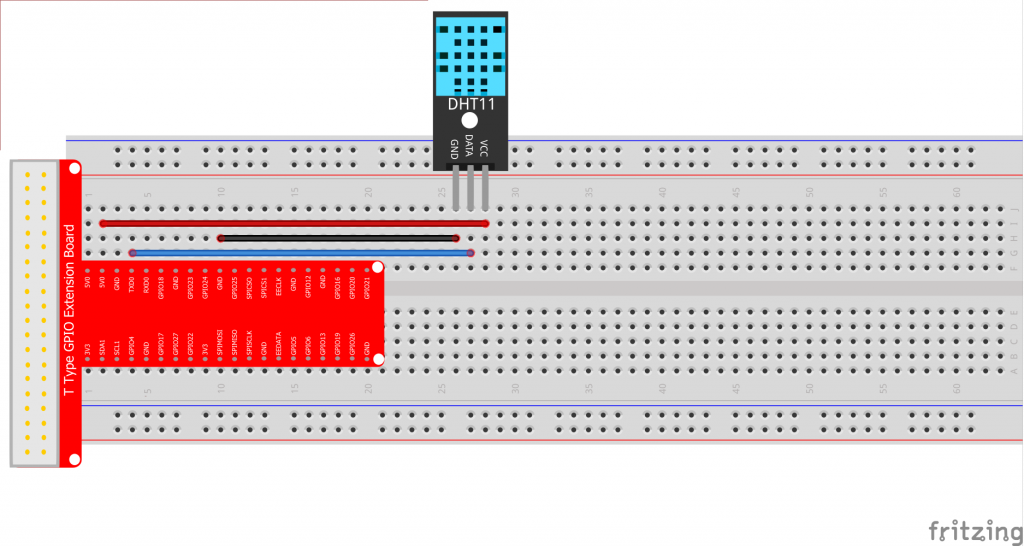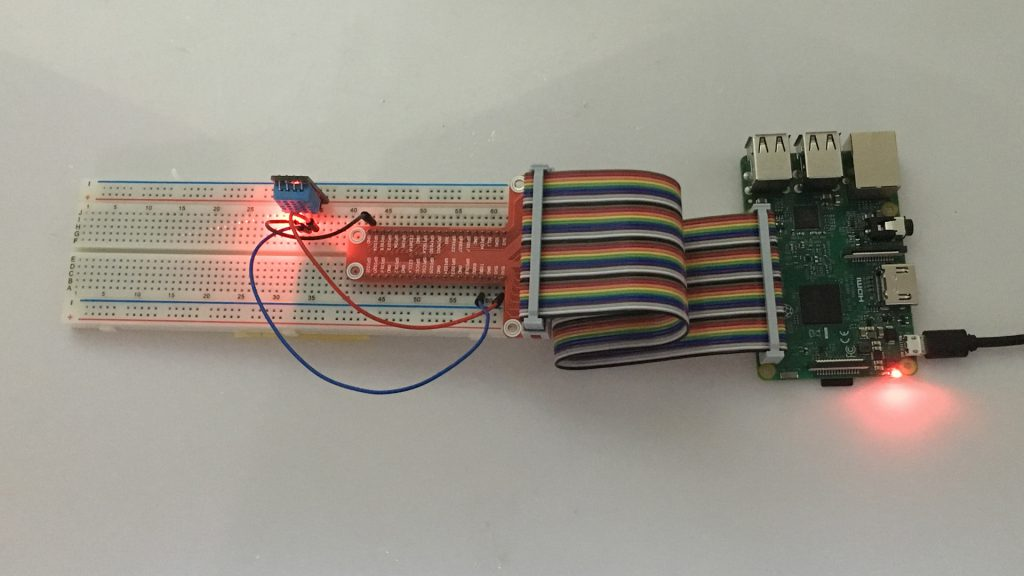Software

We’ll provide two kinds of codes for C language users and Python language users.

For C language users,please take following steps:

Step 1) Download sample code dht11.c from osoyoo.com by typing following commands in terminal:

cd ~

wget http://osoyoo.com/driver/pi3_start_learning_kit_lesson_17/dht11.c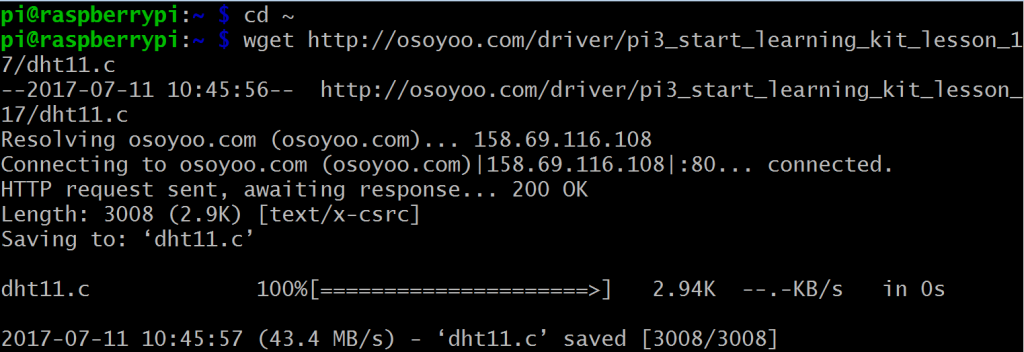Note:
If you want to customize the sample code file , you can use nano editor to edit source code by typing following command in terminal:
sudo  nano dht11.c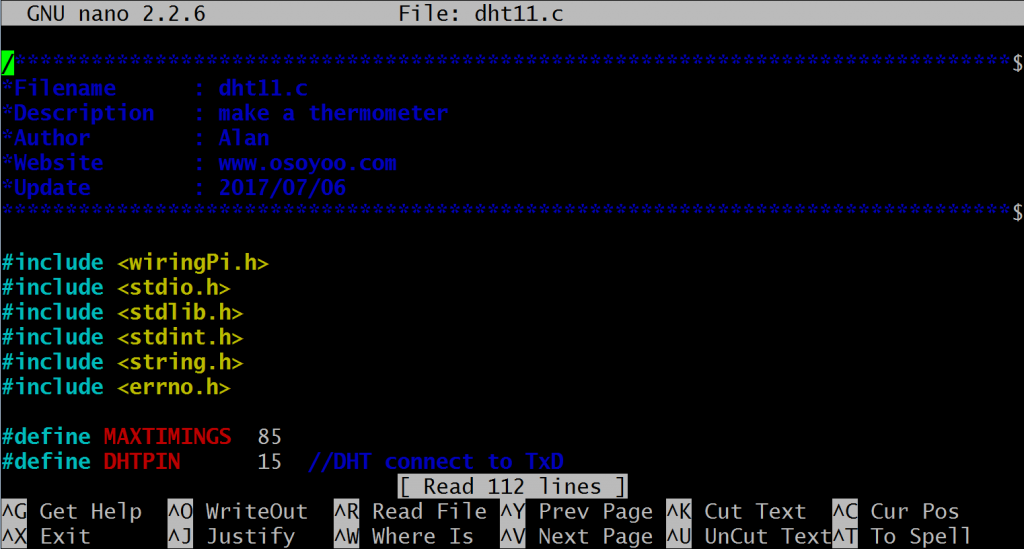3) Compile code

gcc -Wall -o dht11 dht11.c -lwiringPi

4) Run the program

sudo ./dht11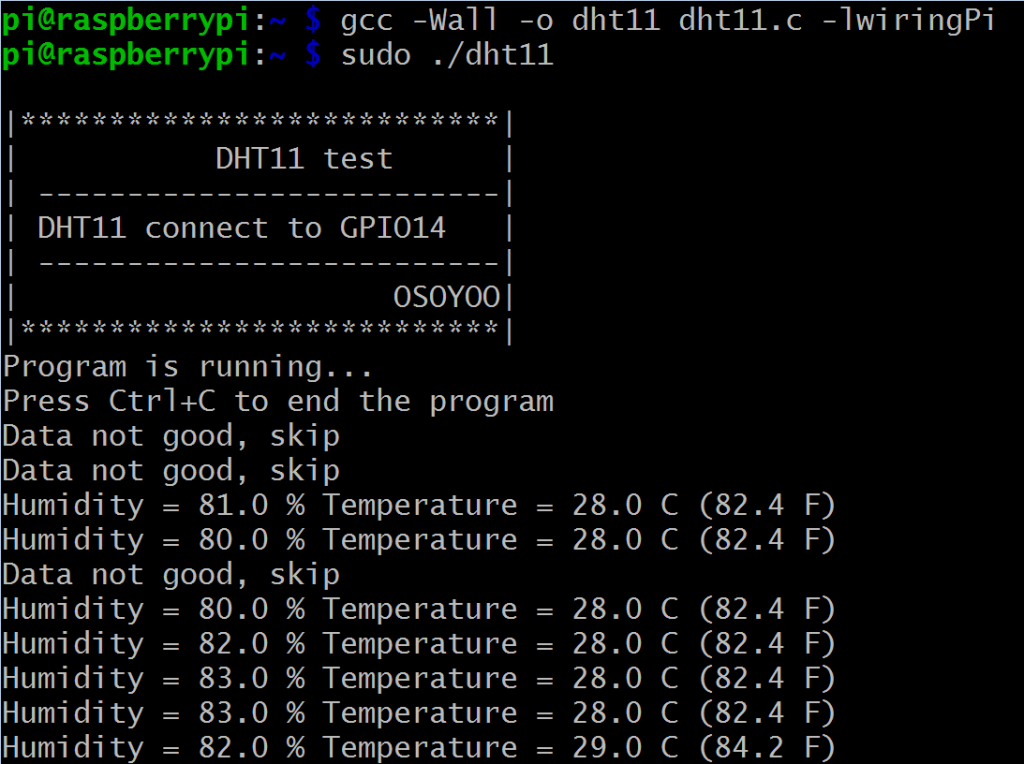5) Program result

Once the program starts running, the terminal will show real temperature and humidity again and again.

C language Sample Code and Explanation comments

``` #include
#include
#include
#include
#include
#include

#define MAXTIMINGS  85
#define DHTPIN      15  //DHT connect to TxD
int dht11_dat ={0,0,0,0,0};//store DHT11 data

{
uint8_t laststate = HIGH;
uint8_t counter = 0;
uint8_t j = 0,i;
float f;//fahrenheit

dht11_dat = dht11_dat = dht11_dat = dht11_dat = dht11_dat = 0;

//pull pin down to send start signal
pinMode( DHTPIN, OUTPUT );
digitalWrite( DHTPIN, LOW );
delay( 18 );
//pull pin up and wait for sensor response
digitalWrite( DHTPIN, HIGH );
delayMicroseconds( 40 );
pinMode( DHTPIN, INPUT );

for ( i = 0; i < MAXTIMINGS; i++ ) { counter = 0; while ( digitalRead( DHTPIN ) == laststate ) { counter++; delayMicroseconds( 1 ); if ( counter == 255 ) { break; } } laststate = digitalRead( DHTPIN ); if ( counter == 255 ) break; //ignore first 3 transitions if ( (i >= 4) && (i % 2 == 0) )
{
//shove each bit into the storage bytes
dht11_dat[j / 8] <<= 1; if ( counter > 16 )
dht11_dat[j / 8] |= 1;
j++;
}
}
//check we read 40 bits(8bit x 5) +verify checksum in the last byte
//print it out if data is good
if ( (j >= 40) &&
(dht11_dat == ( (dht11_dat + dht11_dat + dht11_dat + dht11_dat) & 0xFF) ) )
{
f = dht11_dat * 9. / 5. + 32;
printf( "Humidity = %d.%d %% Temperature = %d.%d C (%.1f F)\n",
dht11_dat, dht11_dat, dht11_dat, dht11_dat, f );
}
else
{
printf( "Data not good, skip\n" );
}
}

void print_info()
{
printf("\n");
printf("|***************************|\n");
printf("|           DHT11 test      |\n");
printf("| --------------------------|\n");
printf("| DHT11 connect to GPIO14   |\n");
printf("| --------------------------|\n");
printf("|                     OSOYOO|\n");
printf("|***************************|\n");
printf("Program is running...\n");
printf("Press Ctrl+C to end the program\n");
}

int main( void )
{
if ( wiringPiSetup() == -1 )
{
fprintf(stderr,"Can't init wiringPi: %s\n",strerror(errno));
exit(EXIT_FAILURE);
}
print_info();
while ( 1 )
{
delay(1000);//wait ls to refresh
}

return(0);
}```

For python users

When programming with Python language , normally we use GPIO library called RPi.GPIO which comes with Rasbian Jessie OS. Click here, you can learn more about RPI.GPIO and Python.

cd ~

wget http://osoyoo.com/driver/pi3_start_learning_kit_lesson_17/dht11.py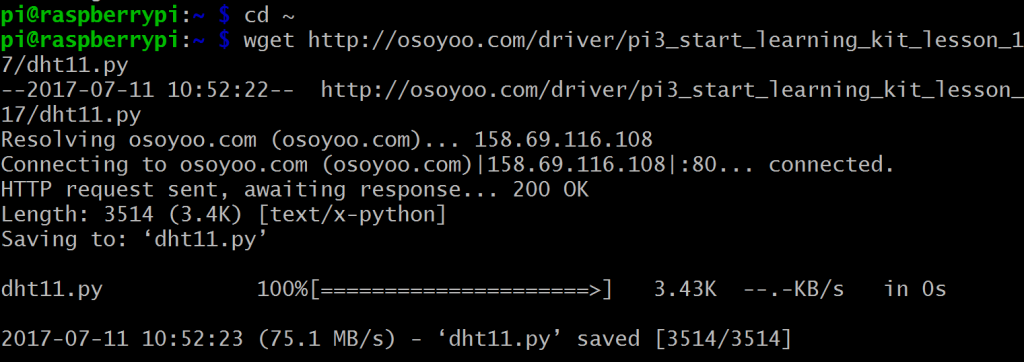Note:
If you want to customize the sample code file , you can use nano editor to edit source code by typing following command in terminal:

sudo nano dht11.py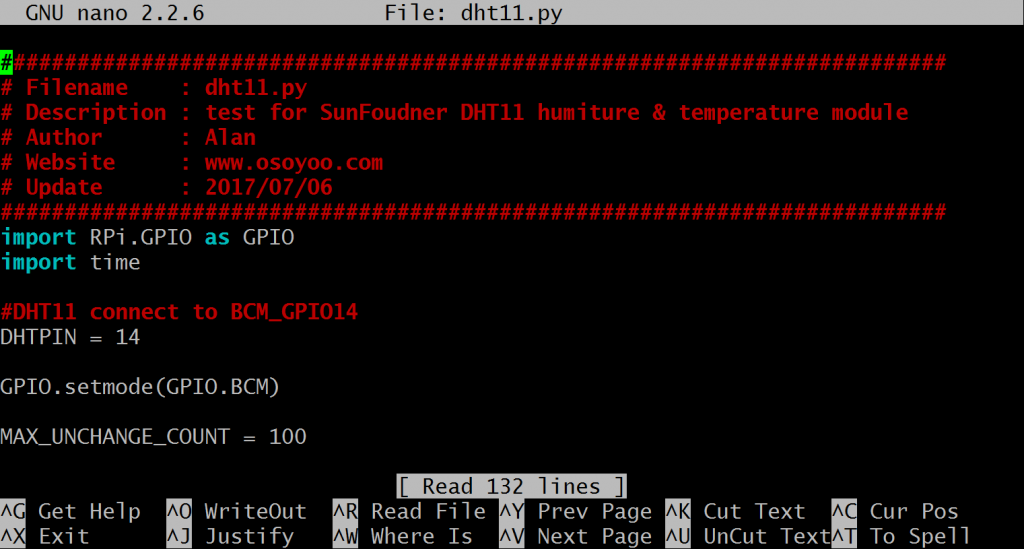3) Run program

sudo python ./dht11.py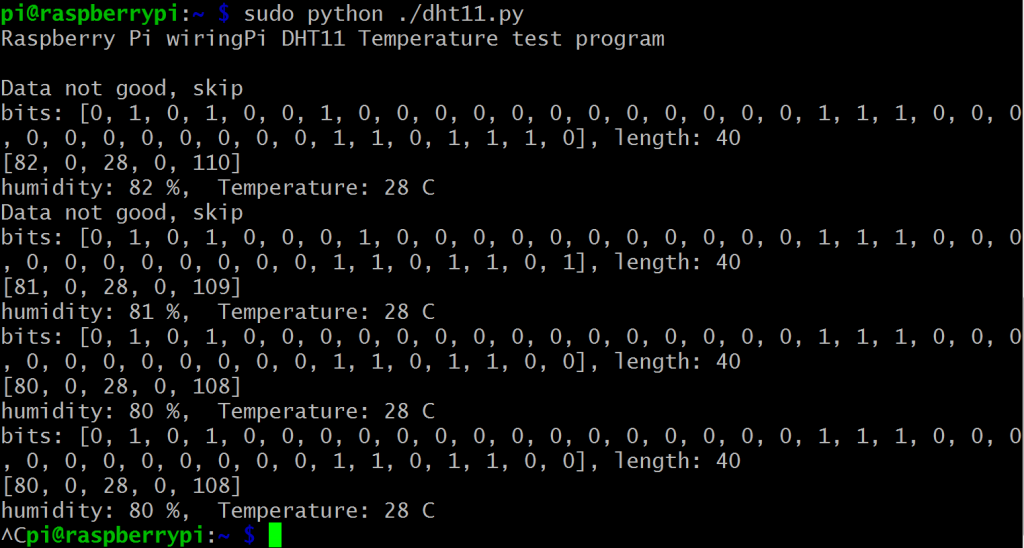4) Program result

Once the program starts running , the terminal will show real temperature and humidity again and again.

Python sample code Analysis:

```import RPi.GPIO as GPIO
import time

#DHT11 connect to BCM_GPIO14
DHTPIN = 14

GPIO.setmode(GPIO.BCM)

MAX_UNCHANGE_COUNT = 100

STATE_INIT_PULL_DOWN = 1
STATE_INIT_PULL_UP = 2
STATE_DATA_FIRST_PULL_DOWN = 3
STATE_DATA_PULL_UP = 4
STATE_DATA_PULL_DOWN = 5

GPIO.setup(DHTPIN, GPIO.OUT)
GPIO.output(DHTPIN, GPIO.HIGH)
time.sleep(0.05)
GPIO.output(DHTPIN, GPIO.LOW)
time.sleep(0.02)
GPIO.setup(DHTPIN, GPIO.IN, GPIO.PUD_UP)

unchanged_count = 0
last = -1
data = []
while True:
current = GPIO.input(DHTPIN)
data.append(current)
if last != current:
unchanged_count = 0
last = current
else:
unchanged_count += 1
if unchanged_count > MAX_UNCHANGE_COUNT:
break

state = STATE_INIT_PULL_DOWN

lengths = []
current_length = 0

for current in data:
current_length += 1

if state == STATE_INIT_PULL_DOWN:
if current == GPIO.LOW:
state = STATE_INIT_PULL_UP
else:
continue
if state == STATE_INIT_PULL_UP:
if current == GPIO.HIGH:
state = STATE_DATA_FIRST_PULL_DOWN
else:
continue
if state == STATE_DATA_FIRST_PULL_DOWN:
if current == GPIO.LOW:
state = STATE_DATA_PULL_UP
else:
continue
if state == STATE_DATA_PULL_UP:
if current == GPIO.HIGH:
current_length = 0
state = STATE_DATA_PULL_DOWN
else:
continue
if state == STATE_DATA_PULL_DOWN:
if current == GPIO.LOW:
lengths.append(current_length)
state = STATE_DATA_PULL_UP
else:
continue
if len(lengths) != 40:
print "Data not good, skip"
return False

shortest_pull_up = min(lengths)
longest_pull_up = max(lengths)
halfway = (longest_pull_up + shortest_pull_up) / 2
bits = []
the_bytes = []
byte = 0

for length in lengths:
bit = 0
if length > halfway:
bit = 1
bits.append(bit)
print "bits: %s, length: %d" % (bits, len(bits))
for i in range(0, len(bits)):
byte = byte << 1 if (bits[i]): byte = byte | 1 else: byte = byte | 0 if ((i + 1) % 8 == 0): the_bytes.append(byte) byte = 0 print the_bytes checksum = (the_bytes + the_bytes + the_bytes + the_bytes) & 0xFF if the_bytes != checksum: print "Data not good, skip" return False return the_bytes, the_bytes def main(): print "Raspberry Pi wiringPi DHT11 Temperature test program\n" while True: result = read_dht11_dat() if result: humidity, temperature = result print "humidity: %s %%, Temperature: %s C" % (humidity, temperature) time.sleep(1) def destroy(): GPIO.cleanup() if __name__ == '__main__': try: main() except KeyboardInterrupt: destroy()```